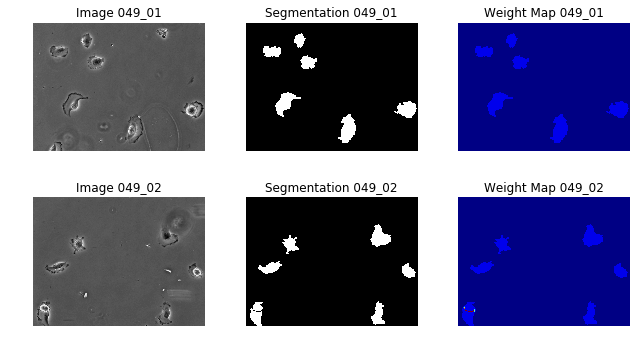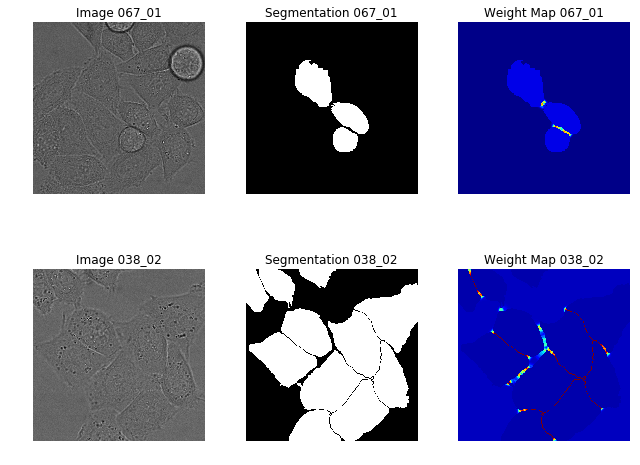# MICCAI Educational Challenge: Using NiftyNet to Train U-Net for Cell Segmentation¶

In this tutorial, you'll learn how to use NiftyNet  to implement the original 2D U-Net. This demo will take you through all the stages of a typical experiment, from data gathering through training, to analysing the results.

## The problem:¶

U-Net  is one of the (if not the) most popular neural net architecture in medical image computing. Its 2015 publication in MICCAI demonstrated a range of world-leading results on a variety of datasets. In this demonstration, we will show you how to use NiftyNet , an open-source platform for deep learning in medical image computing and computer assisted intervention, to train a U-Net and match these results. We also demonstrate how to test various configurations to compare different approaches (for example, which augmentation steps to use).

## Before we start:¶

If you want to run the experiments yourself, you will need to download the appropriate libraries. In addition to NiftyNet, you will need: scikit-image and simpleitk. These are both available as python packages (you can pip install them.

If you don't want to run the experiments yourself, this demo should still be useful as a guide to both U-Net and NiftyNet!

## What's here:¶

If you have any further questions on this, feel free to contact Dr Zach Eaton-Rosen (author of this tutorial).

## The setup:¶

To replicate the paper's results, we have to look at:

## The data:¶

The U-Net paper operated on several different datasets from the "ISBI Cell Tracking Challenge 2015". To get access to these datasets, you can sign up here.

This paper shows results on the "PhC-U373" dataset, and the "DIC-HeLa" dataset.

To run this demonstration as-is, download these datasets and unzip to ./data/u-net. You should see the following folders at this location now:

DIC-C2DH-HeLa
PhC-C2DH-U373

Then run the following command:

python -m demos.unet.file_sorter

to arrange the files in a more convenient way for us.

## The architecture:¶

In terms of implementing the specific u-net architecture, we're in luck! NiftyNet has the 2-d unet implemented already. It's located at niftynet.networks.unet_2d, which you can find by searching the term "unet" at the documentation page of NiftyNet.

## The loss function:¶

U-Net uses a weighted cross-entropy as its loss function. The per-pixel weights are given by a formula which:

1. balances the weights between classes and
2. has an extra term to penalise joining two bits of the segmentation.

$$w(x) = w_c(x) + w_0 . exp \left( -\frac{(d_1(x) + d_2(x))^2}{2\sigma^2} \right)$$

which is equation 2 in the original paper. NiftyNet supports weighted loss functions, but we are going to have to create the weights ourselves. To do that, we can write a python script to generate these weights:

The file I used to make these weights is in demos/unet/make_cell_weights.py

We'll have a look at some of the data here:

In :
import os
import nibabel as nib
import pandas as pd
import numpy as np
import matplotlib.pyplot as plt
import re
import seaborn as sns

%matplotlib inline
def plot_slides(images, figsize=(10,5)):
f, axes = plt.subplots(2,3, figsize=figsize)
for i, slice_id in enumerate(images):
axes[i].imshow(images[slice_id]['img'], cmap='gray')
axes[i].set_title('Image %s' % slice_id)
axes[i].imshow(images[slice_id]['seg'], cmap='gray')
axes[i].set_title('Segmentation %s' % slice_id)
axes[i].imshow(images[slice_id]['weight'], cmap='jet', vmin=0, vmax=10)
axes[i].set_title('Weight Map %s' % slice_id)

for ax in axes[i]:
ax.set_axis_off()
f.tight_layout()

def grab_demo_images(image_dir, slice_ids, image_prefix_dict):
images = {slice_id: {
key: imread(os.path.join(image_dir, image_prefix_dict[key] + '%s.tif' % slice_id))
for key in image_prefix_dict}
for slice_id in slice_ids}
return images



## Visualising the data¶

In :
U373_dir = "../../data/u-net/PhC-C2DH-U373/niftynet_data"
U373_imgs = grab_demo_images(U373_dir, ['049_01', '049_02'], {'img': 'img_', 'seg': 'bin_seg_', 'weight': 'weight_'})

plot_slides(U373_imgs, figsize=(9,5))In :
HeLa_dir = "../../data/u-net/DIC-C2DH-HeLa/niftynet_data/"
HeLa_images = grab_demo_images(HeLa_dir, ['067_01', '038_02'], {'img': 'img_', 'seg': 'bin_seg_', 'weight':'weight_'})

plot_slides(HeLa_images, figsize=(9, 7))These results look very similar to those in Figure 3 of the original paper, so we seem to be on the right track. To do this, I eroded some of the segmentation boundaries to ensure the model learns to predict the gaps between cells, as noted in the U-Net paper.

## Data augmentation:¶

In U-Net, the authors use augmentation via non-linear deformation. Searching the NiftyNet documentation page for deformation, I can see that there is an implementation of elastic deformation in niftynet.layer.rand_elastic_deform module. We will use this augmentation to create variety in our training set, which should improve generalisation to unseen data.

Other things: It can be useful to track the performance on a validation set during training. To do this, NiftyNet uses a dataset_split csv file which allows the data to be labelled as training, validation or evaluation. Because the data for these challenges is quite small, we will use only a few images for validation monitoring.

## The config file:¶

To set all the options, we will use a configuration file. This will include file paths, which network to use, and other options. There is guidance on config files available here.

We will base our configuration files on the config/default_segmentation.ini file in the repository. Here, we go through it step-by-step:

## Data:¶

Here, I show what I've changed from the default example.

############################ input configuration sections
-[modality1]
+[cells]   # you can name this whatever you want
-csv_file=  # we will find the images by searching
-path_to_search = ./example_volumes/monomodal_parcellation
+path_to_search = ./data/u-net/PhC-C2DH-U373/niftynet_data
-filename_contains = T1
+filename_contains = img_
-filename_not_contains =
-spatial_window_size = (20, 42, 42)
+spatial_window_size = (572, 572, 1)
interp_order = 3
-pixdim=(1.0, 1.0, 1.0)
-axcodes=(A, R, S)

[label]
-path_to_search = ./example_volumes/monomodal_parcellation
+./data/u-net/PhC-C2DH-U373/niftynet_data
-filename_contains = Label
+filename_contains = bin_seg_
filename_not_contains =
-spatial_window_size = (20, 42, 42)
+spatial_window_size = (388, 388, 1)
interp_order = 0
-pixdim=(1.0, 1.0, 1.0)
-axcodes=(A, R, S)

+[xent_weights]  # pre-computed cross-entropy weights
+path_to_search = ./data/u-net/PhC-C2DH-U373/niftynet_data
+filename_contains = weight_
+filename_not_contains =
+spatial_window_size = (388, 388, 1)
+interp_order = 3


I've taken out the bits that look relevant, and also added something to load in the weights for the loss function.

The differences: we need to use a loader other than nibabel (I chose skimage, although there are other options). This is to avoid converting the images to nifti before training. We load in binary segmentations for training, although you could also explicitly tell NiftyNet to match all non-zero labels to 1. This way is slightly easier for us to visualise as users, and do appropriate checks.

## System Parameters¶

[SYSTEM]
cuda_devices = ""
num_gpus = 1
-model_dir = ./models/model_monomodal_toy


For the SYSTEM parameters, I increased the number of threads. I removed model_dir, as we will set it via the command line.

## Network¶

[NETWORK]
-name = toynet
+name = unet_2d
-activation_function = prelu
+activation_function = relu
- batch_size = 1
+ batch_size = 4
- decay = 0.1
- reg_type = L2

# volume level preprocessing
+volume_padding_mode = symmetric # this will pad the images with reflection, as per the paper

-# histogram normalisation
-histogram_ref_file = ./example_volumes/monomodal_parcellation/standardisation_models.txt
-norm_type = percentile
-cutoff = (0.01, 0.99)
normalisation = False
-whitening = False
+whitening = True
-normalise_foreground_only=True
+normalise_foreground_only=False
-foreground_type = otsu_plus
-multimod_foreground_type = and

queue_length = 20


I have told the network to use U-Net, and also changed the default normalisation options. I do not want 'foreground' normalisation, as our images take up the whole field of view of the image file. I need the volume padding, because U-Net wouldn't produce segmentation results for voxels near the border otherwise.

## Training¶

[TRAINING]
-sample_per_volume = 32
+sample_per_volume = 2
-rotation_angle = (-10.0, 10.0)
-scaling_percentage = (-10.0, 10.0)
-random_flipping_axes= 1
+random_flipping_axes= 0, 1
-lr = 0.01
+lr = 0.0003
-loss_type = Dice
+loss_type = CrossEntropy
starting_iter = 0
-save_every_n = 100
+save_every_n = 500
-max_iter = 10
+max_iter = 10000
max_checkpoints = 20

+do_elastic_deformation = True
+deformation_sigma = 50
+num_ctrl_points = 6
+proportion_to_deform=0.9

+validation_every_n = 10
+validation_max_iter = 1


The major things I have changed here relate are the loss function and the augmentation. I use the (weighted) cross-entropy, as per the original paper. I also add some instructions for the elastic deformation. It will deform 90% of the images it is passed. Finally, I add an instruction for validation to occur every ten iterations. For the specific deformation parameters, I have determined these by independent experimentation (not shown here).

## Visualising the effects of pre-processing:¶

Here, we are going to visualise the effects of data augmentation (specifically the random elastic deformation) to get some idea of what we should set the parameters to. I'm building this visualisation from the tools in demos/module_examples, where it shows how to visualise the effects of the pre-processing.

Step 1: build a minimal pipeline to see the effects of the augmentation. I will need normalisation (and I remember I used the whitening flag). I will need some padding (again, I chose the volume_padding_mode to be symmetric for this problem). Finally, I will add a RandomElasticDeformationLayer. I will visualise the results of this augmentation and choose a value that seems appropriate. Were the problem one we wanted to heavily optimise, it may be worth cross-validating over parameter choices. Here, however, we just want something that looks reasonable.

In :
import sys
niftynet_path = '../../'
sys.path.append(niftynet_path)

from niftynet.contrib.dataset_sampler.sampler_uniform_v2 import UniformSampler
from niftynet.layer.rand_elastic_deform import RandomElasticDeformationLayer
from niftynet.layer.mean_variance_normalisation import MeanVarNormalisationLayer
from niftynet.layer.rand_flip import RandomFlipLayer

data_param = \
{'cell': {'path_to_search': '../../data/u-net/PhC-C2DH-U373/niftynet_data', # PhC-C2DH-U373, DIC-C2DH-HeLa
'filename_contains': 'img_',
'label': {'path_to_search': '../../data/u-net/PhC-C2DH-U373/niftynet_data', # PhC-C2DH-U373, DIC-C2DH-HeLa
'filename_contains': 'bin_seg_',
'interp_order' : 0}
}

image_name=['cell', 'label'],
border=(92,92,0),
mode='symmetric'))

num_controlpoints=num_controlpoints,
std_deformation_sigma=std_deformation_sigma,
proportion_to_augment=1,
spatial_rank=2))

#                  flip_axes=(0,1)))


INFO:tensorflow:TensorFlow version 1.7.0
['nibabel', 'skimage', 'pillow', 'simpleitk', 'dummy'].
WARNING:niftynet: From /home/zeatonro/anaconda3/envs/sk/lib/python3.6/site-packages/tensorflow/contrib/learn/python/learn/datasets/base.py:198: retry (from tensorflow.contrib.learn.python.learn.datasets.base) is deprecated and will be removed in a future version.
Instructions for updating:
Use the retry module or similar alternatives.

In :
f, axes = plt.subplots(5,4,figsize=(15,15))
f.suptitle('The same input image, deformed under varying $\sigma$')

for i, axe in enumerate(axes):
std_sigma = 25 * i
for ax in axe:
ax.imshow(image_data['cell'].squeeze(), cmap='gray')
ax.imshow(image_data['label'].squeeze(), cmap='jet', alpha=0.1)
ax.set_xticks([])
ax.set_yticks([])
ax.set_title('Deformation Sigma = %i' % std_sigma)

INFO:niftynet:

Number of subjects 230, input section names: ['subject_id', 'cell', 'label']
-- using all subjects (without data partitioning).

INFO:niftynet:

Number of subjects 230, input section names: ['subject_id', 'cell', 'label']
-- using all subjects (without data partitioning).

INFO:niftynet:

Number of subjects 230, input section names: ['subject_id', 'cell', 'label']
-- using all subjects (without data partitioning).

INFO:niftynet:

Number of subjects 230, input section names: ['subject_id', 'cell', 'label']
-- using all subjects (without data partitioning).